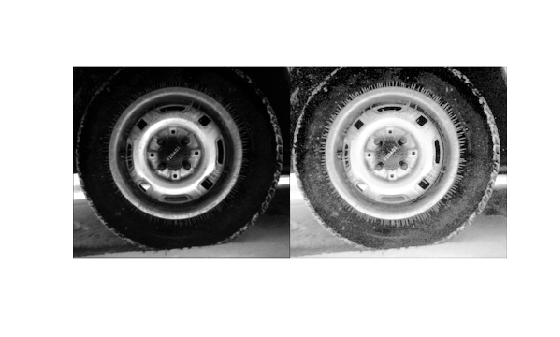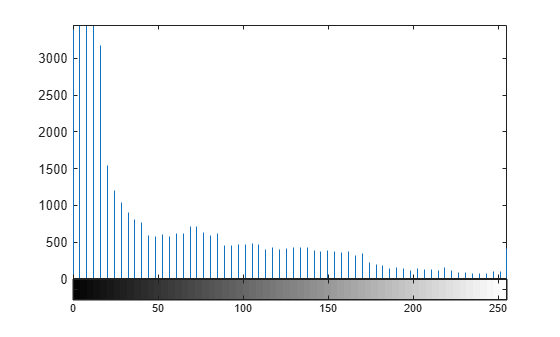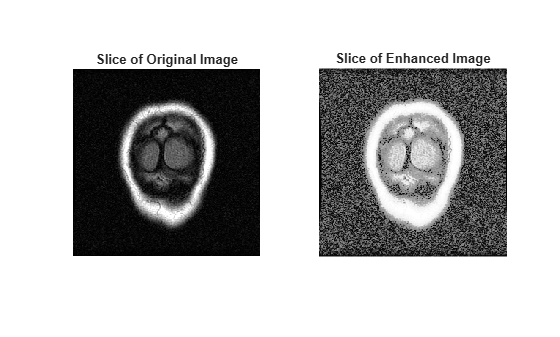# histeq

Enhance contrast using histogram equalization

## Syntax

``````J = histeq(I)``````
``J = histeq(I,n)``
``J = histeq(I,hgram)``
``newmap = histeq(X,map)``
``newmap = histeq(X,map,hgram)``
``````[___,T] = histeq(___)``````

## Description

example

``````J = histeq(I)``` transforms the grayscale image `I` so that the histogram of the output grayscale image `J` has 64 bins and is approximately flat.```
````J = histeq(I,n)` transforms the grayscale image `I` so that the histogram of the output grayscale image `J` with `n` bins is approximately flat. The histogram of `J` is flatter when `n` is much smaller than the number of discrete levels in `I`. ```
````J = histeq(I,hgram)` transforms the grayscale image `I` so that the histogram of the output grayscale image `J` with `length(hgram)` bins approximately matches the target histogram `hgram`.```
````newmap = histeq(X,map)` transforms the values in the colormap so that the histogram of the gray component of the indexed image `X` is approximately flat. The transformed colormap is `newmap`.```
````newmap = histeq(X,map,hgram)` transforms the colormap associated with the indexed image `X` so that the histogram of the gray component of the indexed image (`X`,`newmap`) approximately matches the target histogram `hgram`. The `histeq` function returns the transformed colormap in `newmap`. `length(hgram)` must be the same as `size(map,1)`.```
``````[___,T] = histeq(___)``` also returns the transformation `T` that maps the gray component of the input grayscale image or colormap to the gray component of the output grayscale image or colormap.```

## Examples

collapse all

Read an image into the workspace.

`I = imread('tire.tif');`

Enhance the contrast of an intensity image using histogram equalization.

`J = histeq(I);`

Display the original image and the adjusted image.

```imshowpair(I,J,'montage') axis off```Display a histogram of the original image.

```figure imhist(I,64)```Display a histogram of the processed image.

```figure imhist(J,64)````load mristack`

Perform histogram equalization.

`enhanced = histeq(mristack);`

Display the first slice of data for the original image and the contrast-enhanced image.

```figure subplot(1,2,1) imshow(mristack(:,:,1)) title('Slice of Original Image') subplot(1,2,2) imshow(enhanced(:,:,1)) title('Slice of Enhanced Image')```## Input Arguments

collapse all

Grayscale image, specified as a numeric array of any dimension.

Data Types: `single` | `double` | `int16` | `uint8` | `uint16`

Target histogram, specified as a numeric vector. `hgram` has equally spaced bins with intensity values in the appropriate range:

• [0, 1] for images of class `double` or `single`

• [0, 255] for images of class `uint8`

• [0, 65535] for images of class `uint16`

• [-32768, 32767] for images of class `int16`

`histeq` automatically scales `hgram` so that `sum(hgram)=numel(I)`. The histogram of `J` better matches `hgram` when `length(hgram)` is much smaller than the number of discrete levels in `I`.

Data Types: `single` | `double`

Number of discrete gray levels, specified as a positive integer.

Data Types: `single` | `double`

Indexed image, specified as a numeric array of any dimension. The values in `X` are an index into the colormap `map`.

Data Types: `single` | `double` | `uint8` | `uint16`

Colormap associated with indexed image `X`, specified as a c-by-3 numeric matrix with values in the range [0, 1]. Each row is a three-element RGB triplet that specifies the red, green, and blue components of a single color of the colormap.

Data Types: `double`

## Output Arguments

collapse all

Transformed grayscale image, returned as a numeric array of the same size and class as the input image `I`.

Grayscale transformation, returned as a numeric vector. The transformation `T` maps gray levels in the image `I` to gray levels in `J`.

Data Types: `double`

Transformed colormap, specified as an n-by-3 numeric matrix with values in the range [0, 1]. Each row is a three-element RGB triplet that specifies the red, green, and blue components of a single color of the colormap.

Data Types: `double`

## Algorithms

When you supply a desired histogram `hgram`, `histeq` chooses the grayscale transformation `T` to minimize

`$|{c}_{1}\left(T\left(k\right)\right)-{c}_{0}\left(k\right)|,$`

c0 is the cumulative histogram of the input image `I`, and c1 is the cumulative sum of `hgram` for all intensities k. This minimization is subject to these constraints:

• `T` must be monotonic

• c1(T(a)) cannot overshoot c0(a) by more than half the distance between the histogram counts at a

`histeq` uses the transformation b = T(a) to map the gray levels in `X` (or the colormap) to their new values.

If you do not specify `hgram`, then `histeq` creates a flat `hgram`,

`hgram = ones(1,n)*prod(size(A))/n;`

and then applies the previous algorithm.

## Version History

Introduced before R2006a